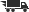Close

# Hierarchical Optimization and Mathematical Physics

• ISBN: 9780792361756
• ISBN10: 079236175X

# Hierarchical Optimization and Mathematical Physics

• List Price: \$199.00
• Binding: Hardcover
• Publish date: 01/01/2000
• ISBN: 9780792361756
• ISBN10: 079236175X
used \$7.72
You save: 96%
new \$150.53
You save: 24%
FREE economy shipping!
Description: This book should be considered as an introduction to a special class of hierarchical systems of optimal control, where subsystems are described by partial differential equations of various types. Optimization is carried out by means of a two-level scheme, where the center optimizes coordination for the upper level and subsystems find the optimal solutions for independent local problems. The main algorithm is a method of iterative aggregation. The coordinator solves the problem with macrovariables, whose number is less than the number of initial variables. On the lower level, we have the usual optimal control problems of mathematical physics, which are far simpler than the initial statements. Thus, we bridge the gap between two disciplines: optimization theory of large-scale systems and mathematical physics. The first motivation was a special model of branch planning, where the final product obeys a precept assortment relation.
Expand description
 Seller: J. Hood, Booksellers, Inc. ★★★★★ ★★★★★ Location: Baldwin City, KS Condition: Good307pp. 307pp. Price: \$28.12 Comments: 307pp. 307pp.